# The TOP - 20 business term deposits16.12.2019

## Bankchart.com.au has surveyed the terms of obtaining business term deposits for 6 months in the national currency. The survey covered all the deposits with any form of interests repayment, regardless of the replenishment option or the deposit partial withdrawal option. Our website's analysts have studied the deposit offers from all Australian banks. Review date - 16.12.2019

As of 01 December 2019 the deposits under review have been offered by 20 banks.  The average interest rate under such deposits equals 1,28 % p.a. The total number of the deposits programs available for the specified terms is 51.

The maximal return among the deposits under review is offered by AMP Bank. The interest rate under "Term Deposit" deposit program constitutes 1,90 % p.a. The table below shows the best offers by each bank selected by Bankchart.com.au (in terms of profitability) for the aforementioned terms.

### The TOP-20 business term deposits for 6 months, as of 01 December 2019

#

Name

Interest Rate

1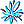AMP Bank

«Term Deposit»

1,90

interest rate, %

rate type

fixed

interest periodicity

at maturity

minimum sum

\$ 25 000,00

2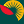Suncorp Bank

1,75

interest rate, %

rate type

variable

interest periodicity

monthly

minimum sum

\$ 0,00

3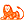ING Direct

1,70

interest rate, %

rate type

fixed

interest periodicity

at maturity

minimum sum

\$ 10 000,00

4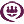RACQ

«Term Deposit»

1,65

interest rate, %

rate type

fixed

interest periodicity

monthly

minimum sum

\$ 1 000,00

5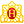Heritage Bank

«Term Deposits»

1,60

interest rate, %

rate type

fixed

interest periodicity

at maturity

minimum sum

\$ 1 000,00

6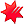National Australia Bank

«Farm Management Deposits»

1,60

interest rate, %

rate type

fixed

interest periodicity

at maturity, annually

minimum sum

\$ 10 000,00

7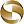Mega International Commercial Bank

1,60

interest rate, %

rate type

fixed

interest periodicity

at maturity

minimum sum

\$ 1 000,00

8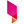Bank Australia

«Fixed term deposits»

1,55

interest rate, %

rate type

fixed

interest periodicity

at maturity

minimum sum

\$ 500,00

9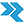Rural Bank

«Fixed rate FMD»

1,55

interest rate, %

rate type

fixed

interest periodicity

at maturity

minimum sum

\$ 5 000,00

10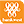Bank of Western Australia

1,55

interest rate, %

rate type

fixed

interest periodicity

at maturity

minimum sum

\$ 10 000,00

11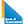Bank of Queensland

«Fixed deposit»

1,55

interest rate, %

rate type

fixed

interest periodicity

at maturity

minimum sum

\$ 5 000,00

12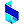Bank of Sydney

«Term Deposit»

1,40

interest rate, %

rate type

fixed

interest periodicity

at maturity

minimum sum

\$ 1 000,00

13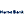Hume Bank

«Term Deposit Account»

1,35

interest rate, %

rate type

fixed

interest periodicity

at maturity, annually

minimum sum

\$ 5 000,00

14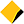Commonwealth Bank of Australia

«Term deposit»

1,35

interest rate, %

rate type

fixed

interest periodicity

at maturity

minimum sum

\$ 50 000,00

15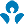Australia and New Zealand (ANZ)

1,25

interest rate, %

rate type

fixed

interest periodicity

quarterly

minimum sum

\$ 0,00

16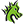St. George Bank

1,20

interest rate, %

rate type

fixed

interest periodicity

at maturity

minimum sum

\$ 1 000,00

17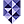Bank of Melbourne

«Fixed Term Deposit»

1,20

interest rate, %

rate type

fixed

interest periodicity

at maturity

minimum sum

\$ 1 000,00

18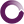Beyond Bank Australia

«monEsaver Account»

1,20

interest rate, %

rate type

variable

interest periodicity

monthly

minimum sum

\$ 0,00

19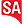Bank SA

«Fixed Term Deposit»

1,20

interest rate, %

rate type

fixed

interest periodicity

at maturity

minimum sum

\$ 1 000,00

20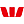Westpac Bank

1,15

interest rate, %

rate type

fixed

interest periodicity

at maturity, annually

minimum sum

\$ 5 000,00

To view the up-to-date rating of the deposits at our website here.

Do you want to know by email when we update the rates?